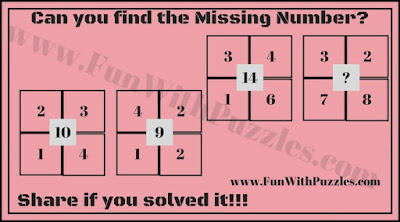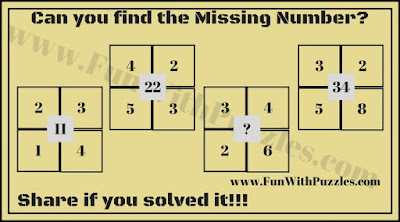Solving the Maths Number Brain Teasers is very much fun. Kids enjoy solving such Fun Maths Puzzles. We have created many fun Maths Picture Puzzles for Kids in which some numbers are given around a Geometrical shape which is logically related to each other. One of the number is missing in the group around the shape and challenge is to find that missing number. Here are some more finding the missing number easy Maths Brain Teasers for kids.
How to solve Fun Maths Brain Teasers
In these Fun Maths Brain Teasers, five numbers are given in a square. These five numbers are related to each other with some logical relationship. One number out of these five number in a square is missing. Your challenge is to find the logical relationship between these numbers and then find the missing number which will replace the question mark.
Answers of these Fun Maths Number Brain Teasers are given at the end along with there explanations.1. Can you find the missing number?2. Can you solve for the missing number?3. What is the missing number?4. Can you find the missing number in this Maths Puzzle?5. Find the missing number in this maths picture puzzle.

Don't forget to check out following Maths Brain Teasers and Puzzles which will twist your mind.
List of Maths Brain Teasers and Puzzles

1. Tough Maths Puzzles Brain Teasers to Tickle your Mind: If you feel that these Fun Maths Number Brain Teasers were easy then do try these Tough Maths Puzzles Brain Teasers which will tickle your mind.

2. Easy Mathematical Puzzle Questions with Answers for students: Time again for Easy Maths Puzzle Questions for Kids. These are easy Maths Puzzles which kids and teens will love to solve.

3. Finding Hidden Faces in the Given Pictures Brain Teasers: Take a break from Maths Brain Teasers and do try visual puzzles which will test your observational skills. Try finding hidden faces in the given picture brain teasers.

4. Fun Brain Teasers For Kids, Teens and Adults with Answers to Challenge your Mind: To try mixed fun brain teasers for kids, teens and adults which will blow your mind.

Answers of these Fun Maths Number Brain Teasers are given as below along with there explanations. To understand the answers and explanations of these Maths Number Brain Teasers lets mark the numbers as A, B, C, D clockwise starting from top left number. Also, mark central number as E.

I)
20
E = A + B + C + D

II)
26
E = (A x C) + (B x D)

III)
4
E = (Difference of A and B) + (Difference of C and D)

IV)
65
E = (Square of A+B) + (Square of C+D)

V)
23
E = ( A x B) + (C + D)